Note: The other languages of the website are Google-translated. Back to English

## Excel Formula: Add hours to time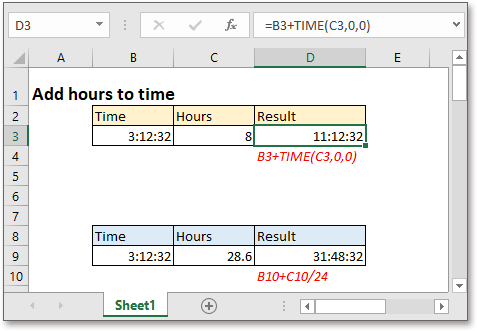In this tutorial, it introduces the formulas to add whole hours or decimal hours to a given time in Excel.

### Add whole hours to time

Generic formula:

 Start_time+TIME(hours,0,0)

Arguments

 Start_time: the time you want to add hours to. Hours: the hours you want to add to time, it must be a positive whole number. If it is negative, the formula returns an error value #NUM!, if the number is a decimal number, such as 6.7, it reckons 6.7 as 6.

Return value

Return a value in time format.

How this formula work

For example, there is a time in cell B3, the number of hours you want to add is displayed in cell C3, please use below formula:

 =B3+TIME(C3,0,0)

Or

 =B3+TIME(8,0,0)

Press Enter key then the result is shown.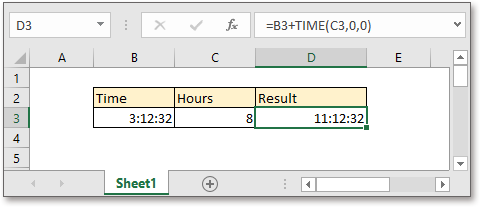Explanation

Generally, TIME function is used to convert decimal numbers (hours, minutes, and seconds) to a time in format hh:mm:ss. Here in this formula, it changes the number of hours (8) to a time (8:00:00), and then the whole formula =B3+TIME(C3,0,0) is calculated to: 3:12:32+8:00:00.

Remark

If you want to subtract the hours from time, please use below formula

 MOD(Start_time+TIME(hours,0,0),1)

For example, there is a time in cell B13, the number of hours you want to subtract is displayed in cell C13, please use below formula:

=MOD(B13-TIME(8,0,0),1)

Or

=MOD(B13-TIME(C13,0,0),1)

Press Enter key then the result is shown.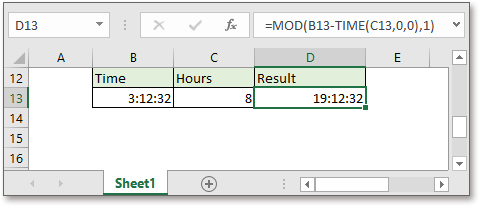Explanation

Generally speaking, it does not allow the negative time in Excel, using the MOD function to flip the negative values to positive will get a correct time.

### Add decimal hours to time

Generic formula:

 Start_time+hours/24

Arguments

 Start_time: the time you want to add hours to. Hours: the hours you want to add to time.

Return value

Return a value in time format.

How this formula work

For example, there is a time in cell B9, the number of hours you want to add is displayed in cell C9, please use below formula:

 =B9+C9/24

Or

 =B9+10/24

Press Enter key then the result is shown.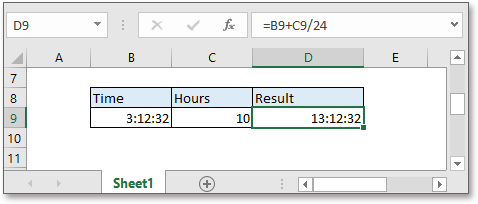Explanation

There are 24 hours a day, and one hour is 1/24 day.

Remark

If you want to subtract the hours from time, please use below formula

 MOD(Start_time-hours/24,1)

For example, there is a time in cell B18, the number of hours you want to subtract is displayed in cell C18, please use below formula:

=MOD(B18-C18/24,1)

Or

=MOD(B18-12/24,1)

Press Enter key then the result is shown.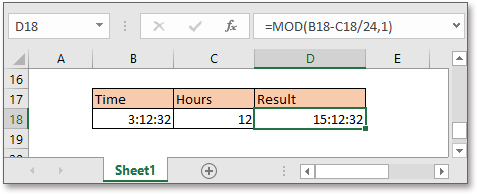Explanation

Generally speaking, it does not allow the negative time in Excel, using the MOD function to flip the negative values to positive will get a correct time.

### Note:

1. The number of hours can be a decimal number while using the formula Start_time+hours/24. See screenshot: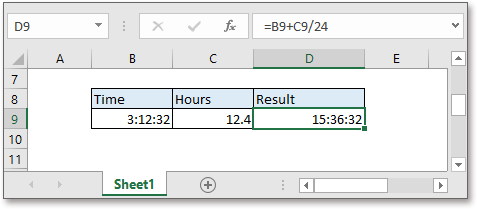2. If the result is over 24 hours, just format the result as [h]:mm:ss format in the Custom section under Number tab of the Format Cells dialog.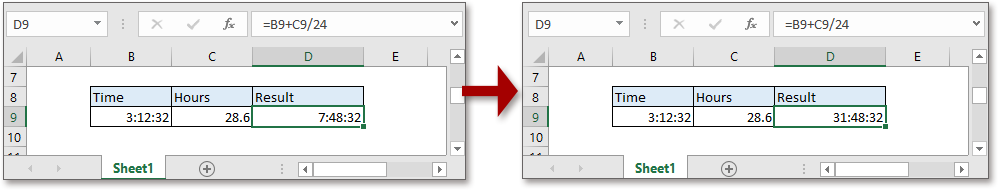### Sample File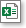Click to download sample file

#### Relative Formulas

• Add Hours Minutes Seconds To Time
Supposing there is a list of time values in Excel, now you want to add 3 hours 45 minutes and 20 seconds to the time values, Excel provides two formulas to help you.
• Extract Or Get Date Only From The Datetime In Excel
To extract only date from a list of datetime cells in Excel worksheet, the INT, TRUNC and DATE functions can help you to deal with this job quickly and easily.
• Get Week Number From Date In Excel
In Excel, how could you get week number from the given date quickly and easily? Normally, the WEEKNUM function can help you to solve this task as quickly as possible.
• Get Work Hours Between Two Dates In Excel
The NETWORKDAYS function in Excel can help you to get the net workdays between two dates, and then multiply the number of working hours per workday to get the total work hours.

#### Relative Functions

• Excel Date Function
DATE function can help us to combine year, month and day numbers from separate cells to a valid date.
• Excel WEEKDAY Function
WEEKDAY function returns an integer number from 1 to 7 to represent the day of the week for a given date in Excel.
• Excel WORKDAY Function
The WORKDAY is used to add a specified number of workdays to a started date and returns the future or past date in a serial number format.
• Excel WORKDAY.INTL Function
WORKDAY.INTL function adds a specified number of workdays to a start date and returns a workday in the future or past.
• Excel YEAR Function
The YEAR function returns the year based on the given date in a 4-digit serial number format.

### The Best Office Productivity Tools

#### Kutools for Excel - Helps You To Stand Out From Crowd

Would you like to complete your daily work quickly and perfectly? Kutools for Excel brings 300 powerful advanced features (Combine workbooks, sum by color, split cell contents, convert date, and so on...) and save 80% time for you.

• Designed for 1500 work scenarios, helps you solve 80% Excel problems.
• Reduce thousands of keyboard and mouse clicks every day, relieve your tired eyes and hands.
• Become an Excel expert in 3 minutes. No longer need to remember any painful formulas and VBA codes.
• 30-day unlimited free trial. 60-day money back guarantee. Free upgrade and support for 2 years.#### Office Tab - Enable Tabbed Reading and Editing in Microsoft Office (include Excel)

• One second to switch between dozens of open documents!
• Reduce hundreds of mouse clicks for you every day, say goodbye to mouse hand.
• Increases your productivity by 50% when viewing and editing multiple documents.
• Brings Efficient Tabs to Office (include Excel), Just Like Chrome, Firefox, And New Internet Explorer.